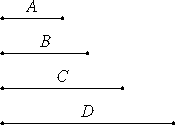# Proposition 23

If four numbers are in continued proportion, and the first is a cube, then the fourth is also a cube.

Let A, B, C, and D be four numbers in continued proportion, and let A be a cube.I say that D is also a cube.

VIII.21

Since between A and D there are two mean proportional numbers B and C, therefore A and D are similar solid numbers. But A is a cube, therefore D is also a cube.

Therefore, if four numbers are in continued proportion, and the first is a cube, then the fourth is also a cube.

Q.E.D.

## Guide

This proposition is used in several propositions in this and the next book starting with VIII.25.